# File format

### Data file

The basic input file is a white-space-delimitered matrix where 0/1/2 denotes the number of one of two alleles.
The rows are SNPs and the columns are individuals (controls first, and then cases).
Genotype data or haplotype data can be used.
Any lines starting with “#” are considred comments in all input files.

Genotype data format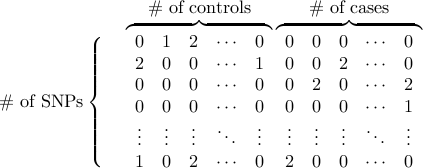Haplotype data format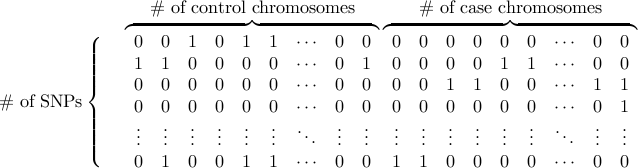Another possible input file format is the covariance band-matrix. Let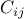be the covariance between the statistic at markerand the statistic at marker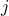. (For many cases including the trend test, allelic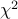test, and Wald test for quantitative traits,turns out to be the correlation coefficient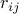between two markers estimated from the dataset.) Suppose we take into account correlation only when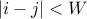(e.g. Window size is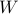). Then, givenmarkers indexed as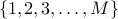, we present the band covariance matrix as the following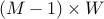rectangular matrix,

Band covariance matrix data format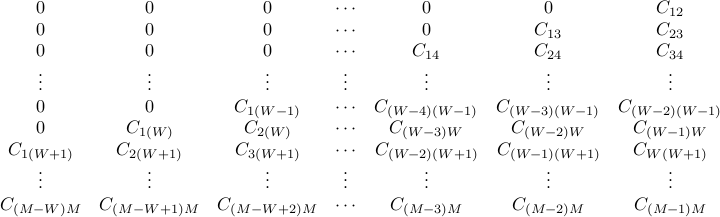Notes
• If the genotype or haplotype format is used, SLIDE runs based on the allelictest or the Armitage’s trend test respectively for case/control studies, and based on the Wald test for quantitative traits.

• Band matrix format allows SLIDE to be applied for a wide variety of statistics, since the user only needs to pre-compute the covariance and provide it.

• For example, in our publication, we describe how to compute the covariance for the Weighted haplotype test and the test for imputed genotypes.

• Previous studies (Seaman and Muller-Myhsok, AJHG2005) also describe the covariance for other statistics such as the transmission disequilibrium test (TDT).

• Note that, if the band matrix format is used, the scaling procedure of SLIDE for higher accuracy will not be used.

Notes regarding unknown genotypes
• In order to maintain the positive semi-definiteness of the correlation matrix, the unknown genotypes need to be resolved beforehand.

• The simplest way is to randomly assign missing allele based on the allele frequency. The format converter (PED -> SLIDE) we provide below automatically performs this random asignment.

• Another possible way is to assign the allele frequency itself to the allele as a floating point number. Although SLIDE currently does not accept genotype/haplotype format including floating point number, SLIDE accepts the band covariance matrix file, therefore one can compute the covariance based on the assigned floating point number and use it as input to SLIDE.

• Yet another possibility is to use available imputation algorithm. After imputation, one can compute the band covariance matrix based on the posterior probabilties of alleles using the derivation described in our publication, and use it as input to SLIDE.

• If the number of unknown genotypes is very small compared to the number of known genotypes, the currently implemented approach (random assignment based on frequency) will provide a sufficiently accurate multiple testing correction.

### MAP file (optional)

PLINK-format MAP file is optional to display rsid in output.

### Example files

example1.slide.gz (5.4Mb)

Genotype data format/ 5,754 SNPs/ 2,934 controls/ 1,928 cases/ mimicing a chr 22 data of a 500K chip

example2.slide.gz (230Mb)

Haplotype data format/ 203,689 SNP/ 4,000 control chromosomes/ 4,000 case chromosomes/ mimicing a chr 1 data of 2.7 million HapMap SNPs

example3.bandcovmat.gz (5.6Mb)

Band covariance matrix format/ 5,754 SNPs/ window size 100

# Basic usage

Case/control study
```./slide [-G|-H] [data file] [#control] [#case] [w] [tmpdir] [#sampling] [seed] [mapfile(option)]
```
• Parameters

• -G is for genotype data and -H is for haplotype data
(Results will be based on the trend test and thetest respectively).

• #control and #case are the numbers of control and case individuals (or chromosomes if -H is used).

• w is the window size defined in terms of the number of SNPs.
SLIDE will take into account correlations between SNPs only within this window size (100 is enough for most cases).

• tmpdir is a temporary storage directory for intermediate files.

• #sampling is the number of sampling iterations which can be (roughly) thought of as the number of permutations.

• seed is the random number generation seed.

• Output

• tmpdir/slide.pvalues containing corrected p-values.

• Usage example

• slide -G example1.slide 2934 1928 100 mydir 10000 12345678

• Approximate time

• ~2 m (example1.slide) and ~1 h (example2.slide) for 10,000 sampling with w=100

Use 4-step advanced usage for the followings
1. Tests for quantitative traits.

2. Band covariance matrix input data format.

3. Utilize a cluster of computer processors.

4. Split the genome into chromosomes for easier genome-wide analysis.

SLIDE is composed of four programs. (The executable slide for basic usage is nothing more than a wrapper script running these four.)

1. slide_1prep : data pre-processing.

2. slide_2run : run the actual sampling.

3. slide_3sort : sort the maximum statistic.

4. slide_4correct : correct p-values.

## Step 1

Case/control study
```./slide_1prep [-G|-H] [data file] [#control] [#case] [w] [prep file]
```
Quantitative traits
```./slide_1prep [-QG|-QH] [data file] [#individual] [w] [prep file]
```
For band covariance matrix
```./slide_1prep [-C] [band cov mat file] [w] [prep file]
```
• Parameters

• Case/control study

• -G is for genotype data and -H is for haplotype data
(Results will be based on the trend test and thetest respectively).

• #control and #case are the numbers of control and case individuals (or chromosomes if -H is used).

• Quantitative traits

• -QG is for genotype data and -QH is for haplotype data
(Results will be based on the Wald test).

• #individual is the numbers of individuals (or chromosomes if -QH is used).

• The trait file is not needed because we make an assumption that the statistic will follow the normal distribution after a random permutation.

• w is the window size defined in terms of the number of SNPs.
SLIDE will take into account correlations between SNPs only within this window size (100 is enough for most cases).

• For band matrix, w has to be the same window size used for constructing the band matrix.

• [prep file] is any temporary file name you want to use as a storage file.

• Output

• Many pre-processed files that look like [prep file].xxxxxx

• Usage example

• ./slide_1prep -G example1.slide 2934 1928 100 mydir/myprepfile

• Approximate time

• ~1 m (example1.slide) and ~0.5 h (example2.slide) with w=100

## Step 2

```./slide_2run [prep file] [max stat file] [#sampling] [seed]
```
• Parameters

• Use the same [prep file] that you specified in step 1.

• Output

• [max stat file] storing the sampled maximum statistics over the markers.

• Usage example

• ./slide_2run mydir/myprepfile mydir/mymaxstat 10000 12345678

• Approximate time

• ~1 m (example1.slide) and ~0.5 h (example2.slide) with 10,000 sampling and w=100

• If you do a large number (e.g. 1,000,000) of sampling, you might want to use a cluster.

Using cluster
• Suppose we run 10,000 samplings to create each of 100 max-statistic files,
maxstat1, maxstat2, …, maxstat100,
using 100 CPUs (of course, using different seeds).
Each file only contains binary floats written by the C program.

• Thus, by merging them as follows,
cat maxstat* > merged_maxstat
we can obtain a merged result file equivalent to 1,000,000 samplings.

For Genome-wide studies
• If you divide the whole genome into chromosomes, repeat step 1 and step 2 for each chromosome.

## Step 3

```./slide_3sort [sorted file] [max stat file(chr1)] [max stat file(chr2)] [max stat file(chr3)] ...
```
• Parameters

• [max stat file] is the output of step 2.

• If you divided genomic region into N independent regions (e.g. chromosomes), provide N max-stat-files. In that case, the number of sampling for each file has to be identical.

• If you did not divide the region, just use one max-stat-file.

• Output

• [sorted file] stores the sorted maximum statistics over the whole genome.

• Usage example:

• ./slide_3sort mydir/mysortedstat mydir/mymaxstat

## Step 4

### P-value correction

To correct pointwise p-values,

```./slide_4correct -p [sorted file] [pointwise-p file] [final output file] [mapfile(optional)]
```
• Parameters

• [sorted file] is the output of step 3.

• [pointwise-p file] is a text file containing pointwise p-values you want to correct, delimitered by space or newline.

• Note that if you used -H or -G option, for each chromosome, step 1 creates a pointwise p-value file which looks like [prep file].txt_pointwisep.
You can use this file to correct p-values of every SNP in each chromosome.

• You can also specify MAP file to see rsid in output. In that case, the number of SNPs in MAP file has to be the same as the number of pointwise p-values to correct.

• Usage example:

• ./slide_4correct -p mydir/mysortedstat mydir/myprepfile.txt_pointwisep mydir/finaloutput

• Output

• [final output file] containing corrected p-values, which will look like

Example output
```#Result based on 10000000 sampling
#SNP_id Pointwise-P     Corrected-P     Approx.STDEV(% of P)    Effective#ofTests       Note
SNP1    1.16801500e-07  4.24000000e-04  6.51014765e-06(1.53541%)        3630
SNP2    2.02511400e-07  7.27500000e-04  8.52625794e-06(1.17199%)        3592
SNP3    3.43213500e-07  1.22440000e-03  1.10584847e-05(0.90318%)        3567
SNP4    5.75063100e-07  2.05320000e-03  1.43142739e-05(0.69717%)        3570
SNP5    9.61198000e-07  3.41440000e-03  1.84465224e-05(0.54026%)        3552
SNP6    1.61919700e-06  5.69580000e-03  2.37978105e-05(0.41781%)        3518
SNP7    2.74274700e-06  9.52860000e-03  3.07210120e-05(0.32241%)        3474
SNP8    4.56832800e-06  1.57566000e-02  3.93806165e-05(0.24993%)        3449
SNP9    7.73045900e-06  2.63954000e-02  5.06938683e-05(0.19206%)        3414
SNP10   1.30862400e-05  4.38772000e-02  6.47703569e-05(0.14762%)        3353
```
• SNP-id will indicate rsid if MAP file is provided.

• STDEV is the standard deviation of the corrected p-value estimate, which needs a caution to interpret. If STDEV is very large, then SLIDE’s corrected p-value may not be accurate due to stochastic error. However, even if STDEV is extremely small (e.g.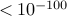), it does not mean that SLIDE’s corrected p-value is that extremely near to the true p-value, because SLIDE may converge to a p-value that differs from the true p-value slightly by 1-2% (which is still accurate).

• If the corrected p-value is extremely close to 1 because the maximum statistic is always more significant than the pointwise p-value, SLIDE just reports the corrected p-value 1 and STDEV 0 (although this can be mathematically misleading, these SNPs are anyhow not of major interest.)

### Per-marker threshold estimation

Alternatively, you may want to find the per-marker threshold for the significance threshold of .05 or .01.
To obtain per-marker thresholds,

```./slide_4correct -t [sorted file] [sig threshold file] [final output file]
```
• Parameters

• [sig threshold file] is a text file containing significance threshold you want to obtain per-marker threshold, delimitered by space or newline.

### Interactive modes

There are interactive modes as well,

```./slide_4correct -ip [sorted file] [pointwise-p]
./slide_4correct -it [sorted file] [sig threshold]
```
• Usage example:

• ./slide_4correct -ip mydir/mysortedstat 0.0000001
0.05432
./slide_4correct -it mydir/mysortedstat 0.05
0.00000008345

# Format converter

A format converter from PED format to SLIDE format is provided in the SLIDE package.
If you have ped and map files named PEDFILE.ped and PEDFILE.map,

```./ped2slide [PEDFILE] [output file]
```

writes SLIDE format to [output file]